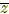index: click on a letter A B C D E F G H I J K L M N O P Q R S T U V W X Y Z A to Z index index: subject areas numbers & symbols sets, logic, proofs geometry algebra trigonometry advanced algebra & pre-calculus calculus advanced topics probability & statistics real world applications multimedia entrieswww.mathwords.com about mathwords website feedback

Complex Conjugate

The complex conjugate of a + bi  is abi, and similarly the complex conjugate of abi  is a + bi. This consists of changing the sign of the imaginary part of a complex number. The real part is left unchanged.

Complex conjugates are indicated using a horizontal line over the number or variable. For example,.

Note: Complex conjugates are similar to, but not the same as, conjugates.

ExpressionComplex Conjugate5 – 2i 4i + 1 –5i 125 + 2i –4i + 1 5i 12